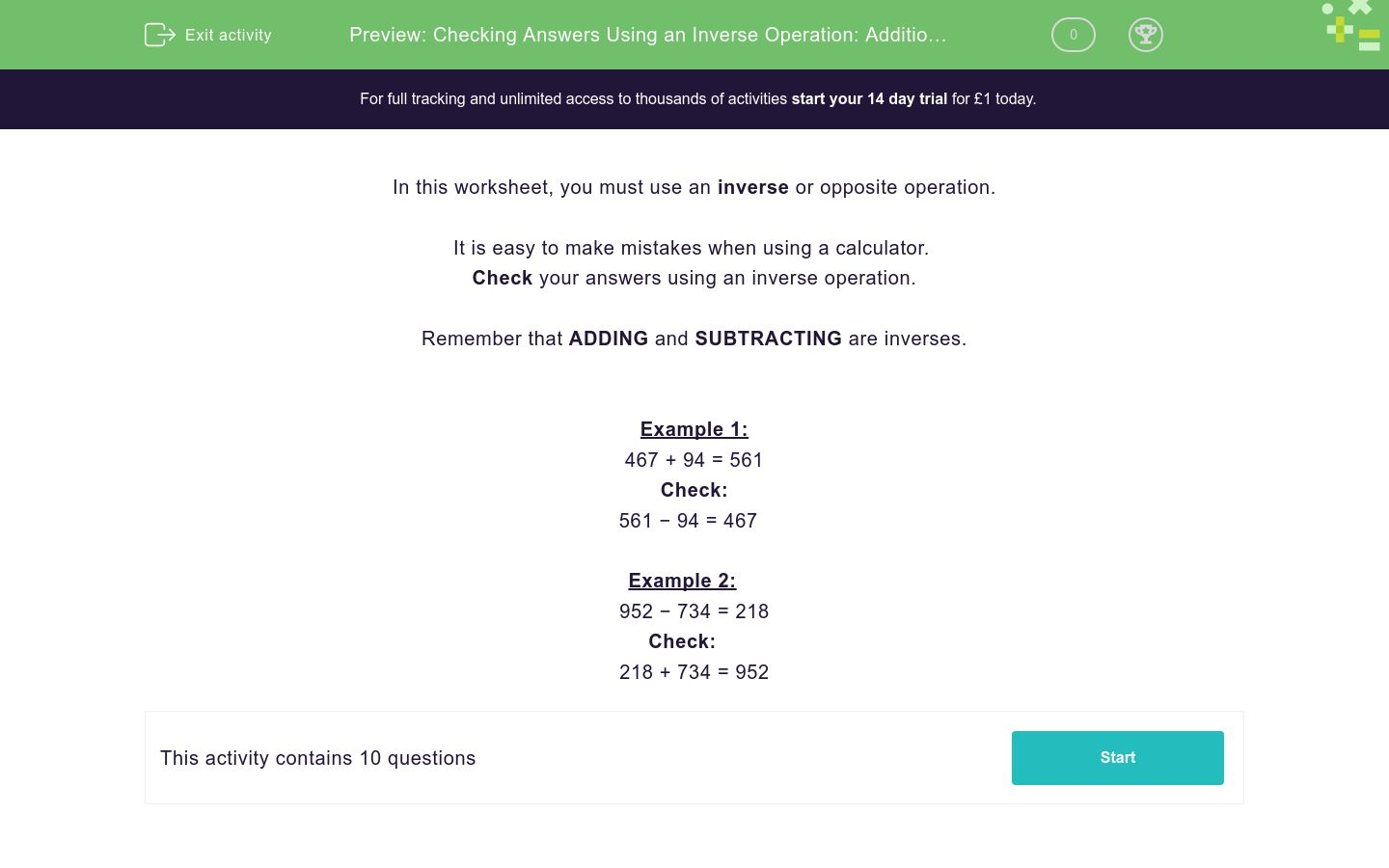### Affordable pricing from £10/month

In this worksheet, students check answers using an inverse operation.Key stage:  KS 3

Curriculum topic:   Number

Curriculum subtopic:   Use Relationships Between Operations

Difficulty level:### QUESTION 1 of 10

In this worksheet, you must use an inverse or opposite operation.

It is easy to make mistakes when using a calculator.

Remember that ADDING and SUBTRACTING are inverses.

Example 1:

467 + 94 = 561

Check:

561 − 94 = 467

Example 2:

952 − 734 = 218

Check:

218 + 734 = 952

Check the answer using an inverse operation for the given operation.

938 + 120 = 1058
1058 − 120 =

Check the answer using an inverse operation for the given operation.

938 − 120 = 818
818 + 120 =

Check the answer using an inverse operation for the given operation.

804 + 353 = 1157
1157 − 353 =

Check the answer using an inverse operation for the given operation.

804 − 353 = 451
451 + 353 =

Check the answer using an inverse operation for the given operation.

571 + 405 = 976
976 − 405 =

Check the answer using an inverse operation for the given operation.

571 − 405 = 166
166 + 405 =

Check the answer using an inverse operation for the given operation.

742 + 473 = 1215
1215 − 473 =

Check the answer using an inverse operation for the given operation.

742 − 473 = 269
269 + 473 =

Check the answer using an inverse operation for the given operation.

350 + 33 = 383
383 − 33 =

Check the answer using an inverse operation for the given operation.

350 − 33 = 317
317 + 33 =
• Question 1

Check the answer using an inverse operation for the given operation.

938 + 120 = 1058
1058 − 120 =
938
• Question 2

Check the answer using an inverse operation for the given operation.

938 − 120 = 818
818 + 120 =
938
• Question 3

Check the answer using an inverse operation for the given operation.

804 + 353 = 1157
1157 − 353 =
804
• Question 4

Check the answer using an inverse operation for the given operation.

804 − 353 = 451
451 + 353 =
804
• Question 5

Check the answer using an inverse operation for the given operation.

571 + 405 = 976
976 − 405 =
571
• Question 6

Check the answer using an inverse operation for the given operation.

571 − 405 = 166
166 + 405 =
571
• Question 7

Check the answer using an inverse operation for the given operation.

742 + 473 = 1215
1215 − 473 =
742
• Question 8

Check the answer using an inverse operation for the given operation.

742 − 473 = 269
269 + 473 =
742
• Question 9

Check the answer using an inverse operation for the given operation.

350 + 33 = 383
383 − 33 =
350
• Question 10

Check the answer using an inverse operation for the given operation.

350 − 33 = 317
317 + 33 =
350
---- OR ----

Sign up for a £1 trial so you can track and measure your child's progress on this activity.

### What is EdPlace?

We're your National Curriculum aligned online education content provider helping each child succeed in English, maths and science from year 1 to GCSE. With an EdPlace account you’ll be able to track and measure progress, helping each child achieve their best. We build confidence and attainment by personalising each child’s learning at a level that suits them.

Get started